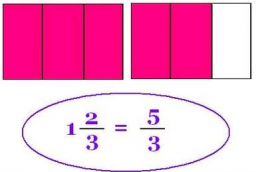# Assignment 68494

Write the assignment in a fraction.
Fill in the number in the box so that the equality applies:
(Write the result as a fraction in the base form.)
(1 1/3 - 3/4) · ___ = 1/2

x =  6/7

### Step-by-step explanation:Did you find an error or inaccuracy? Feel free to write us. Thank you!

Tips to related online calculators
Need help to calculate sum, simplify or multiply fractions? Try our fraction calculator.
Do you have a linear equation or system of equations and looking for its solution? Or do you have a quadratic equation?